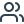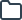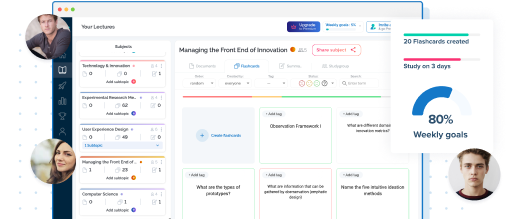### Select your language

Suggested languages for you:# Lernmaterialien für Machine Learning an der Universität Düsseldorf

Greife auf kostenlose Karteikarten, Zusammenfassungen, Übungsaufgaben und Altklausuren für deinen Machine Learning Kurs an der Universität Düsseldorf zu.

TESTE DEIN WISSEN

What means "modus ponens", what "modus tollens"?

Lösung anzeigen
TESTE DEIN WISSEN

modus ponens: A=>B, A=1 => B=1

modus tollens: A=>B, B=0 => A=0

Lösung ausblenden
TESTE DEIN WISSEN

Dram the Machine Learning Matrix

Lösung anzeigen
TESTE DEIN WISSEN

supv.                  unsupv.

disc.     classif.               cluster.

cont.    regress.              dim.reduct.

Lösung ausblenden
TESTE DEIN WISSEN

Name Kolmogorov axioms.

Lösung anzeigen
TESTE DEIN WISSEN

- 0 <= p(A) <= 1
- P(Omega) = 1
- p(A) + p(B) = p(A,B) if A and B are mutually exclusive

Lösung ausblenden
TESTE DEIN WISSEN

What is  clustering?

Lösung anzeigen
TESTE DEIN WISSEN

This is building up classes or finite cluster without a correcting instance even without predefined beliefs or classes

Lösung ausblenden
TESTE DEIN WISSEN

What is meant with classification?

Lösung anzeigen
TESTE DEIN WISSEN

Classification means that data is associated to finite predefined classes and there is a correcting instance that ensures correct association.

Lösung ausblenden
TESTE DEIN WISSEN

What means PCA?

Lösung anzeigen
TESTE DEIN WISSEN

PCA is a dimensionality reduction process e.g. Eigenfaces.

Lösung ausblenden
TESTE DEIN WISSEN

What implies p(B|A)= 1?

Lösung anzeigen
TESTE DEIN WISSEN

p(B|A) = 1, modus ponens
p(not B|not A) = 1 modus tollens
p(B|not A) <= p(B)
p(A|B) >= p(A)

Lösung ausblenden
TESTE DEIN WISSEN

Write down Bayes Rule and label all parts of it.

Lösung anzeigen
TESTE DEIN WISSEN

p(H|data) = p(data|H)p(H)/p(data)

posterior belief = likelyhood * prior belief / evidence

Lösung ausblenden
TESTE DEIN WISSEN

What differs deductive from plausible  reasoning?

Lösung anzeigen
TESTE DEIN WISSEN

Deductive reasoning is derived from mathematical logic , while plausible reasoning describes that a Possibility can become more or less plausible instead of true or false only.

Lösung ausblenden
TESTE DEIN WISSEN

Name the tools of machine learning with each one example.

Lösung anzeigen
TESTE DEIN WISSEN

-probability theory e.g bayes rule
-linear algebra e.g y=ax+n
-optimization e.g minimize f(x)
-computer science e.g programming

Lösung ausblenden
TESTE DEIN WISSEN

What is meant with regression?

Lösung anzeigen
TESTE DEIN WISSEN

Means that the data is labeled to continuous functions, that shows the distribution of values e.g. prediction of prices

Lösung ausblenden
TESTE DEIN WISSEN

Name four subclasses of machine learning.

Lösung anzeigen
TESTE DEIN WISSEN

-supervised learning
-semi-supervised learning
-unsupervised learning
-reinforcement learning

Lösung ausblenden•263207 Karteikarten
•3439 Studierende
•126 Lernmaterialien

## Beispielhafte Karteikarten für deinen Machine Learning Kurs an der Universität Düsseldorf - von Kommilitonen auf StudySmarter erstellt!

Q:

What means "modus ponens", what "modus tollens"?

A:

modus ponens: A=>B, A=1 => B=1

modus tollens: A=>B, B=0 => A=0

Q:

Dram the Machine Learning Matrix

A:

supv.                  unsupv.

disc.     classif.               cluster.

cont.    regress.              dim.reduct.

Q:

Name Kolmogorov axioms.

A:

- 0 <= p(A) <= 1
- P(Omega) = 1
- p(A) + p(B) = p(A,B) if A and B are mutually exclusive

Q:

What is  clustering?

A:

This is building up classes or finite cluster without a correcting instance even without predefined beliefs or classes

Q:

What is meant with classification?

A:

Classification means that data is associated to finite predefined classes and there is a correcting instance that ensures correct association.

Q:

What means PCA?

A:

PCA is a dimensionality reduction process e.g. Eigenfaces.

Q:

What implies p(B|A)= 1?

A:

p(B|A) = 1, modus ponens
p(not B|not A) = 1 modus tollens
p(B|not A) <= p(B)
p(A|B) >= p(A)

Q:

Write down Bayes Rule and label all parts of it.

A:

p(H|data) = p(data|H)p(H)/p(data)

posterior belief = likelyhood * prior belief / evidence

Q:

What differs deductive from plausible  reasoning?

A:

Deductive reasoning is derived from mathematical logic , while plausible reasoning describes that a Possibility can become more or less plausible instead of true or false only.

Q:

Name the tools of machine learning with each one example.

A:

-probability theory e.g bayes rule
-linear algebra e.g y=ax+n
-optimization e.g minimize f(x)
-computer science e.g programming

Q:

What is meant with regression?

A:

Means that the data is labeled to continuous functions, that shows the distribution of values e.g. prediction of prices

Q:

Name four subclasses of machine learning.

A:

-supervised learning
-semi-supervised learning
-unsupervised learning
-reinforcement learning### Erstelle und finde Lernmaterialien auf StudySmarter.

Greife kostenlos auf tausende geteilte Karteikarten, Zusammenfassungen, Altklausuren und mehr zu.

## Das sind die beliebtesten StudySmarter Kurse für deinen Studiengang Machine Learning an der Universität Düsseldorf

Für deinen Studiengang Machine Learning an der Universität Düsseldorf gibt es bereits viele Kurse, die von deinen Kommilitonen auf StudySmarter erstellt wurden. Karteikarten, Zusammenfassungen, Altklausuren, Übungsaufgaben und mehr warten auf dich!

## Das sind die beliebtesten Machine Learning Kurse im gesamten StudySmarter Universum

##### 3. Machine Learning

FOM Hochschule für Oekonomie & Management

##### Machine Learning (FS21)

University of Basel

## Die all-in-one Lernapp für Studierende

##### Greife auf Millionen geteilter Lernmaterialien der StudySmarter Community zu##### Erstelle Karteikarten und Zusammenfassungen mit den StudySmarter Tools